Elements of Mathematica Programs

Mathematica can be used in the same fashion as a traditional programming language, such a fortran or C.  For  frequently used programs or computationally intensive applications, Mathematica is not the best programming language choice, but it can be very effective as a way to test out ideas or generate a single numerical result.

Evaluating a sequence of instrutions (;;;)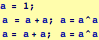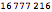Note above that line breaks without ";" are points where the instructions get executed. This three-line sequence has five executions and produces two lines of output and the instructions get executed sequentially.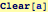Loops

In Mathematica, it is generally not a good idea to use looping constructions such as Do, For, and While. The preferred method to construct and operate on lists---however, it is ok to use looping constructions as beginners.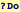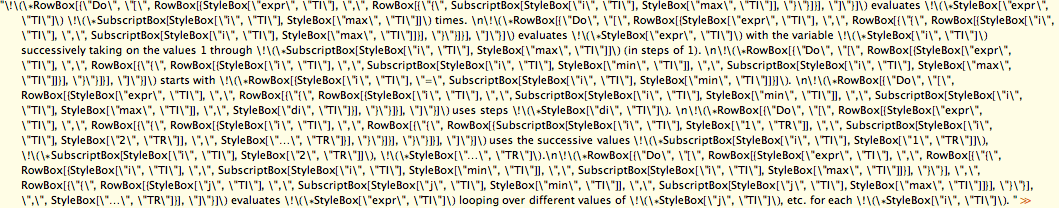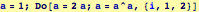In this case, Do does not produce screen output. The following will and is a simple illustration of how formatted output can be programmed: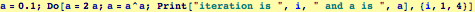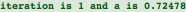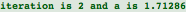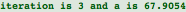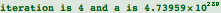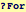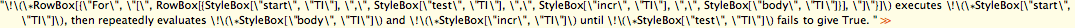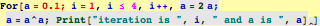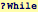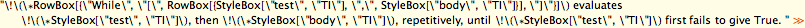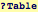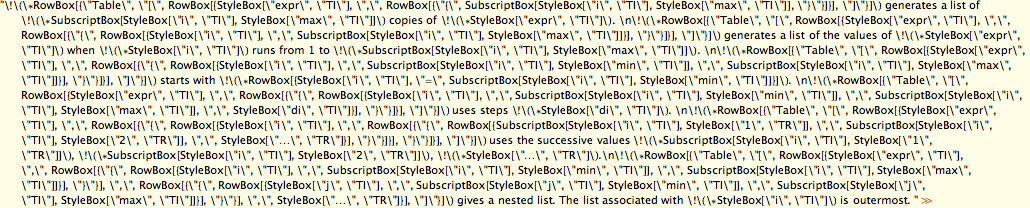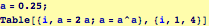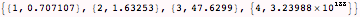Note above that we have asked Mathematica to create a list of two-element lists...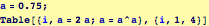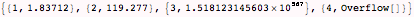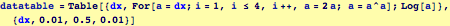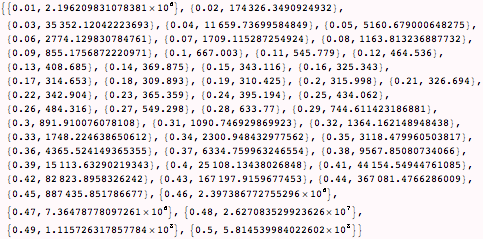In the instruction above, note that the variable "datatable" is a list. In Mathematica, variables can be numbers, expressions, lists, plots, ...  We will see that this feature is very useful.